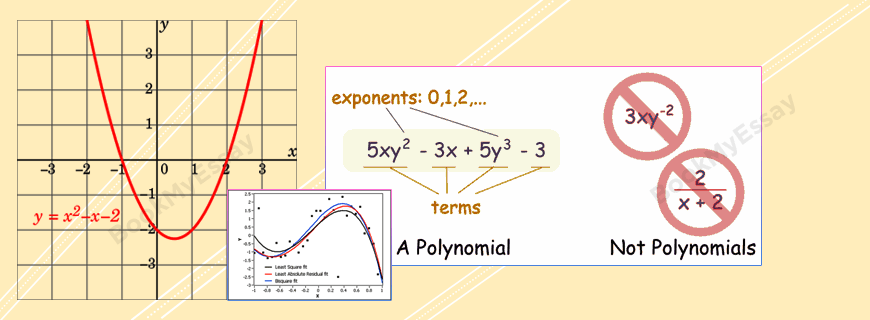# Polynomial Assignments HelpGet a Free Quote
It's free and always will be.
Page - +
words
NWL

## Polynomial Assignment Help

A polynomial is a very important mathematical expression consisting of a sum of n-number of terms. Each term in a polynomial expression contains a variable term raised to a certain power and multiplied by a coefficient. The simplest form of polynomial has one variable. One such simplest form of polynomial contains looks like the following: anxn an-1xn-1 + … + a2x2 + a1x1 + ax. BookMyEssay is one of the most trusted assignment help that provides best mathematical tutors and experts for Polynomial assignment help and writing service.

Feature of Polynomial Experts

The experts associated with BookMyEssay are all possessing higher degrees including Ph.D. in mathematics, and also possessing years of experience in mathematics assignment writing. Problems on polynomials are not easy to solve because a number of formulas and methods are involved in solving such problems. Apart from solving these assignments, the writers also take care of other aspects of assignments on polynomials.

### Characteristics of Polynomials

• When a number is added or subtracted with another number, that is called ‘term‘.
• When the numbers are multiplied with each other, they are called factors.
• A variable of a polynomial is an expression that can change under different conditions. Like an expression 5x2 + 1, if x = 7 then the value of the expression will be 246. Again, if x = 6, then the value will be 181.
• Polynomials also contains constant values along with the variables. Thus, in the expression, 5x2 + 1 the constant terms are 5 and 1. So, with the changing values of x, the expression will go on changing. Again, the equation y = ax+ bx + c, contains a, b, and c as constants.
• The degree of a term is the sum of all the exponents of all the variables in a term. So in the equation y = ax+ bx + c, the term ax2 is possessing a degree of 2 and the tern bx is possessing a degree of 1.
• The leading term of a polynomial is the term of highest degree. Thus in the expression 5x2 + 1, the leading term is 5x2. Similarly, the leading coefficient is the coefficient of the leading term.
• The degree of a polynomial is the degree of the leading term.
• The constant term of a polynomial expression is the term with no variable. Like in the expression 5x2 + 1, the constant term is 1.
• The polynomial function possesses the following format: y = ax+ bx + c
• Domain – Unless otherwise mentioned, the domain of a polynomial is always ranges from negative infinity to positive infinity. Again, there are other forms of polynomials where the limit of the variables may be clearly defined, like the polynomial f(x) = x2, −3 ≤ x ≤ 3. Here the limit of the variable is clearly restricted.
• In a polynomial equation of the form P(x) = 5x3− 4x2 + 7x − 8 = 0, the values of the variable x, for which the equation remains valid, are called the roots of the equation.
• A polynomial equation can also be represented in graphical form too. In such case, the roots of the equation will intercept the x-axis and y-axis.

There are multiple of other characteristics of polynomial, which are equally important to understand the properties of the polynomial and students need to have detail understanding on all these characteristics of polynomial to solve wide many types of problems on this topic. But, it is really hard to grasp the topic and it’s all types of problems within a few months’ time period. Thus polynomial assignment writing not always smooth sailing for students of higher mathematics.

Examiners always like to assign the toughest tasks to students, which may not be always solvable for all students. In such situations, it is feasible to take help with Polynomial assignment writing from BookMyEssay and hire an expert to solve the problems and submit the assignment within the deadlines.

### Services Provided by BookMyEssay

The mathematics experts associated with BookMyEssay for helping the students facing difficulty in solving polynomial assignment and polynomial homework writing are highly skilled people. They have deep knowledge on mathematics. Hence, they know, how to approach an assignment on polynomial and how to complete a really impressive assignment. Moreover, these writers ensure –

• 100% adherence to the guidelines.
• 100% customized assignments.
• 100% plagiarism free assignments.

The entire Polynomial Assignment help is a very affordable custom assignment writing service for all kinds of mathematics students studying in any country around the world. Besides, the team of experts and student help desk executives are completely dedicated to the service in such a way that thousands of students have obtained the highest marks in polynomial assignments after getting help from BookMyEssay.